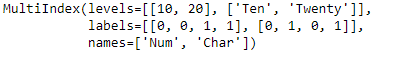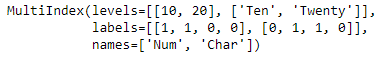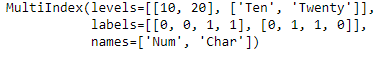# Python | Pandas MultiIndex.set_labels()

• Last Updated : 22 Sep, 2021

Python is a great language for doing data analysis, primarily because of the fantastic ecosystem of data-centric python packages. Pandas is one of those packages and makes importing and analyzing data much easier.
Pandas MultiIndex.set_labels() function set new labels on MultiIndex. Defaults to returning new index.

Syntax: MultiIndex.set_labels(labels, level=None, inplace=False, verify_integrity=True)
Parameters :
labels : new labels to apply
level : level(s) to set (None for all levels)
inplace : if True, mutates in place
verify_integrity : if True, checks that levels and labels are compatible
Returns: new index (of same type and class…etc)

Example #1: Use MultiIndex.set_labels() function to reset the labels of the MultiIndex.

## Python3

 `# importing pandas as pd``import` `pandas as pd` `# Create the MultiIndex``midx ``=` `pd.MultiIndex.from_tuples([(``10``, ``'Ten'``), (``10``, ``'Twenty'``),``                                  ``(``20``, ``'Ten'``), (``20``, ``'Twenty'``)],``                                       ``names ``=``[``'Num'``, ``'Char'``])` `# Print the MultiIndex``print``(midx)`

Output :Now let’s reset the labels of the MultiIndex.

## Python3

 `# resetting the labels the MultiIndex``midx.set_labels([[``1``, ``1``, ``0``, ``0``], [``0``, ``1``, ``1``, ``0``]])`

Output :As we can see in the output, the labels of the MultiIndex has been reset.

Example #2: Use MultiIndex.set_labels() function to reset any specific label only in the MultiIndex.

## Python3

 `# importing pandas as pd``import` `pandas as pd` `# Create the MultiIndex``midx ``=` `pd.MultiIndex.from_tuples([(``10``, ``'Ten'``), (``10``, ``'Twenty'``),``                                  ``(``20``, ``'Ten'``), (``20``, ``'Twenty'``)],``                                        ``names ``=``[``'Num'``, ``'Char'``])` `# Print the MultiIndex``print``(midx)`

Output :Now let’s reset the ‘Char’ label of the MultiIndex.

## Python3

 `# resetting the labels the MultiIndex``midx.set_labels([``0``, ``1``, ``1``, ``0``], level ``=``'Char'``)`

Output :As we can see in the output, the ‘Char’ label of the MultiIndex has been reset to the desired value.

My Personal Notes arrow_drop_up